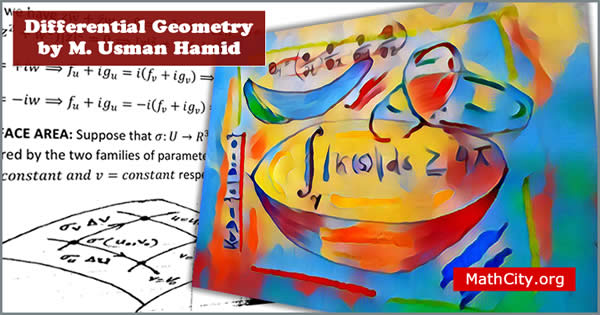# Differential Geometry by M Usman HamidThese notes are initially provided by Mr. Anwar Khan. Later send by many persons. We are really very thankful to all for providing these notes and appreciates their effort to publish these notes on MathCity.org

It covers the one section of the syllabus of Complex Analysis paper of MSc Mathematics. Also it is teaches as complete subject in semester system. See the contents of the notes given below to see the topics covered by these notes.

The study of geometry by using the method of calculus is called Differential Geometry (DG). it is the study of curves, surfaces and their abstract generalization. The study of DG required two primary tools; Linear Algebra and Calculus

 Name Differential Geometry Prof. Muhammad Usman Hamid Mr. Anwar Khan 126 pages PDF (see Software section for PDF Reader) 3.5 mB
• Review of vectors and scalars
• Curves with torsion
• Global properties of curves
• Differential geometry of surfaces
• Open set
• Continuous mapping
• Homeomorphism
• Surface
• One parameter family of surfaces
• Characteristic of a surface
• Level surface
• Principal curvatures, principal sections, principal directions and lines of curvature

• notes/differential-geometry-m-usman-hamid